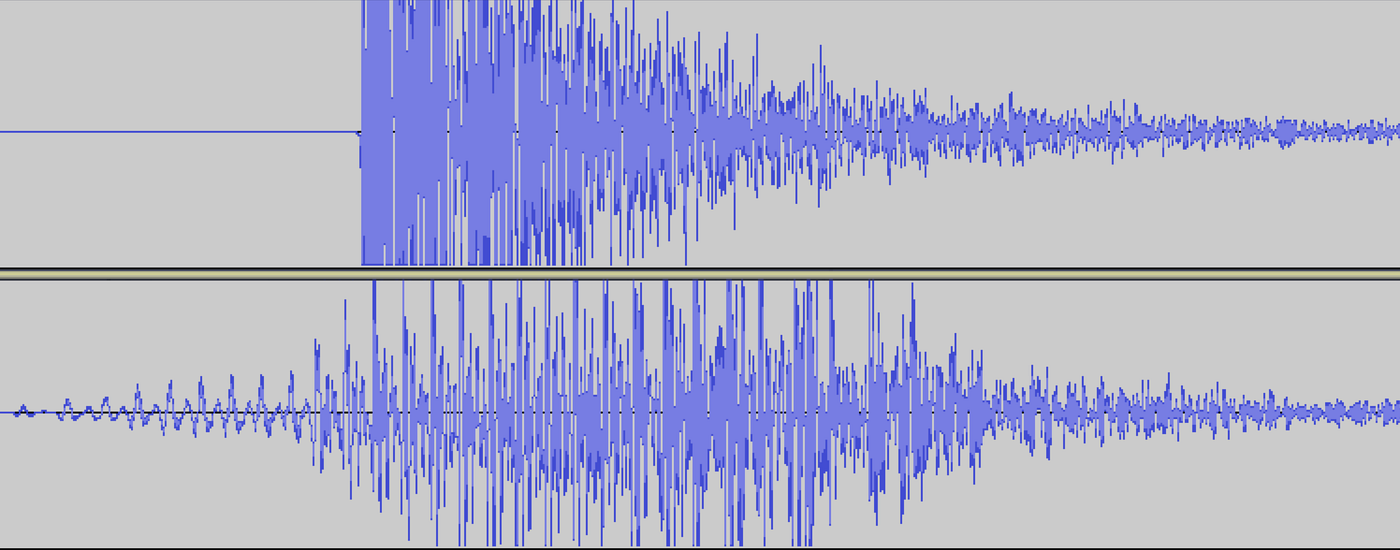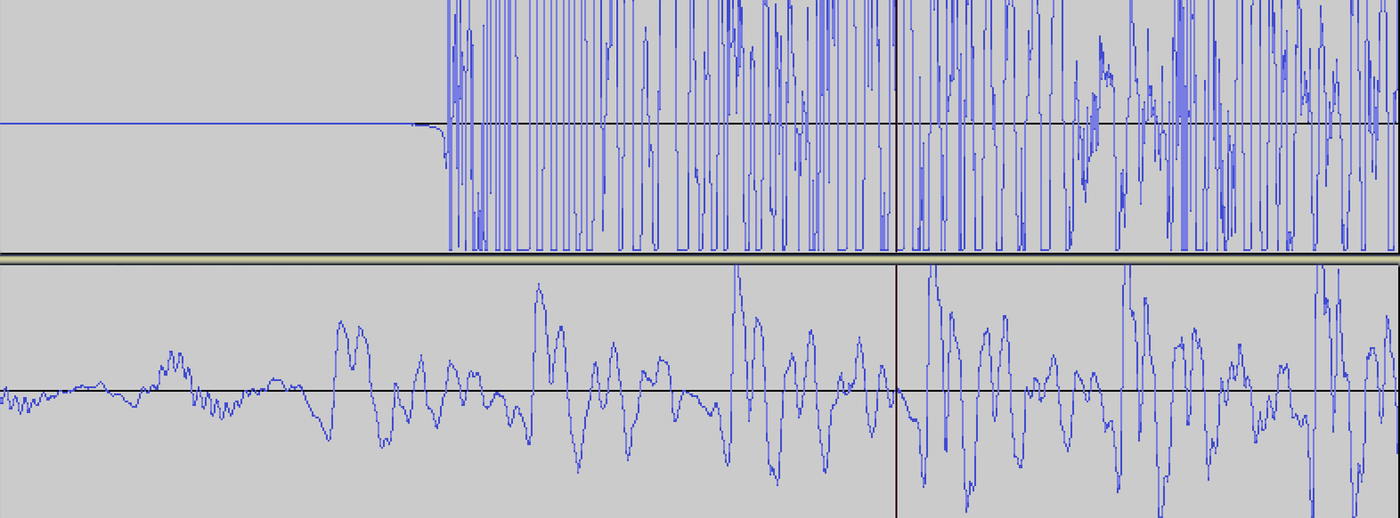# Introduction

`int irPin = 11; void setup() {   Serial.begin(9600);   pinMode(irPin, INPUT); }`
`int commandMask = 127;void loop() {  duration = pulseIn(irPin, LOW);  if (duration >= 2400) { // start burst    int bitnum = 0;    int bitstring = 0;    int duration = pulseIn(irPin, LOW);     while (duration >= 600 && duration < 2000) {      int currbit = 0;      if (abs(duration-1200)<abs(duration-600))        currbit = 1;       bitstring = (currbit << bitnum) | bitstring;      ++bitnum;      duration = pulseIn(irPin, LOW);    }    int address = bitstring >> 7;    int command = bitstring & commandMask;    Serial.print(“Address: “);    Serial.print(address);    Serial.print(“, Command: “);    Serial.println(command);  }}`

# Transmitting the Remote Commands

`const int startBurstLength = 2400;const int oneBurstLength = 1200;const int zeroBurstLength = 600;const int waitLength = 600;const byte topVal = 200;const byte dutyCycle = topVal/4;`
`void setup() {  Serial.begin(9600);  pinMode(3, OUTPUT);}`
`void loop() {  if (Serial.available()) {    command = Serial.readString().toInt();    if (command == 0) return;     for (int i=0; i<3; i++) {      transmit(command);      delay(40);    }  }}`
`void mark(int delayUs) {  TCCR2A |= (1<<COM2B1);  delayMicroseconds(delayUs);  space(waitLength);}void space(int delayUs) {  TCCR2A &= ~(1<<COM2B1); // disable output on b  delayMicroseconds(delayUs);}void sendTVAddress() {  mark(oneBurstLength);  for (char i=0; i<4; i++) {    mark(zeroBurstLength);  }}void sendCommand(byte command) {  TIMSK2 &= ~(1<<TOIE2);   digitalWrite(3, LOW);   OCR2A = topVal;  OCR2B = dutyCycle;  TCCR2A = (1<<WGM20);  TCCR2B = (1<<WGM22) | (1<<CS20);  mark(startBurstLength);   for (char i=0; i<7; i++) {    if (command % 2 == 0) mark(zeroBurstLength);    else mark(oneBurstLength);    command >>= 1;  }}void transmit(byte command) {  sendCommand(command);  sendTVAddress();}`
`void mark(int delayUs) {  TCCR2A |= (1<<COM2B1);  delayMicroseconds(delayUs);  space(waitLength);}`
`void space(int delayUs) {  TCCR2A &= ~(1<<COM2B1); // disable output on b  delayMicroseconds(delayUs);}`

# Counting Claps

`int micPin = A0;int micVal;void setup() {  Serial.begin(9600);   pinMode(micPin, INPUT);}void loop() {  micVal = analogRead(micPin);  Serial.println(micVal);}`(Top) A recording of a clap. (Bottom) A recording of a shoutA clap’s attack is much shorter than anything the human voice can produce
`int micPin = A0;int micVal;int threshold = 620;unsigned int nClaps = 0;unsigned long lastClapEnd;int clapTimeout = 500;int clapMax;unsigned long currTime;unsigned long start;int timeToClapMax;int clapMaxThreshold = 630;int maxAttackTime = 10;void setup() {  Serial.begin(9600);  pinMode(micPin, INPUT);}void getClapMaxInfo() {  start = millis();  clapMax = micVal;  timeToClapMax = 0;  currTime = millis();  while (currTime-start < 100) {    micVal = analogRead(micPin);    if (micVal > clapMax) {      clapMax = micVal;      timeToClapMax = currTime-start;    }    currTime = millis();  }}void waitUntilBelowThreshold() {  micVal = analogRead(micPin);  while (micVal > threshold) {    delay(10);    micVal = analogRead(micPin);  }}boolean isClap() {  return clapMax > clapMaxThreshold && timeToClapMax <= maxAttackTime;}void loop() {  micVal = analogRead(micPin);   if (micVal > threshold) {    getClapMaxInfo();     if (isClap())      ++nClaps;    waitUntilBelowThreshold();    lastClapEnd = millis();   } else {    if (nClaps > 0 && millis()-lastClapEnd >= clapTimeout) {      Serial.println(nClaps);      nClaps = 0;    }  }}`

# Putting it All Together

`#include <IRremote.h>const int numCommands = 5;int nClaps2Command[numCommands] = {0x90, 0x890, 0xa90, 0x490, 0xc90};int times2Repeat[numCommands] = {1, 1, 1, 5, 5};IRsend irsend;... other global variables`
`void transmit(int command, int repeat) {  for (char n=0; n<repeat; n++) {    for (char i=0; i<3; i++) {      irsend.sendSony(command, 12);      delay(40);  }  }}`
`void loop() { ...   if (isClap()) {     ++nClaps;     if (nClaps > numCommands)        nClaps = 0;   }   ...  } else {    if (nClaps > 0 && millis()-lastClapEnd >= clapTimeout) {      ...      transmit(nClaps2Command[nClaps-1], times2Repeat[nClaps-1]);    }  }}`